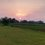# Newton sums in easier notation

$\LARGE a^n+b^n+c^n=(a+b+c)(a^{n-1}+b^{n-1}+c^{n-1})-(ab+bc+ac)(a^{n-2}+b^{n-2}+c^{n-2})+abc(a^{n-3}+b^{n-3}+c^{n-3})$

$\huge a^n+b^n=(a+b)(a^{n-1}+b^{n-1})-ab(a^{n-2}+b^{n-2})$

$\huge a^n+\frac{1}{a^n}=(a+\frac{1}{a})(a^{n-1}+\frac{1}{a^{n-1}})-(a^{n-2}+\frac{1}{a^{n-2}})$

For exercises: Try this problem

Try this very nice set by Aditya.

And thisNote by Sanjeet Raria
5 years, 10 months ago

This discussion board is a place to discuss our Daily Challenges and the math and science related to those challenges. Explanations are more than just a solution — they should explain the steps and thinking strategies that you used to obtain the solution. Comments should further the discussion of math and science.

When posting on Brilliant:

• Use the emojis to react to an explanation, whether you're congratulating a job well done , or just really confused .
• Ask specific questions about the challenge or the steps in somebody's explanation. Well-posed questions can add a lot to the discussion, but posting "I don't understand!" doesn't help anyone.
• Try to contribute something new to the discussion, whether it is an extension, generalization or other idea related to the challenge.

MarkdownAppears as
*italics* or _italics_ italics
**bold** or __bold__ bold
- bulleted- list
• bulleted
• list
1. numbered2. list
1. numbered
2. list
Note: you must add a full line of space before and after lists for them to show up correctly
paragraph 1paragraph 2

paragraph 1

paragraph 2

[example link](https://brilliant.org)example link
> This is a quote
This is a quote
    # I indented these lines
# 4 spaces, and now they show
# up as a code block.

print "hello world"
# I indented these lines
# 4 spaces, and now they show
# up as a code block.

print "hello world"
MathAppears as
Remember to wrap math in $$ ... $$ or $ ... $ to ensure proper formatting.
2 \times 3 $2 \times 3$
2^{34} $2^{34}$
a_{i-1} $a_{i-1}$
\frac{2}{3} $\frac{2}{3}$
\sqrt{2} $\sqrt{2}$
\sum_{i=1}^3 $\sum_{i=1}^3$
\sin \theta $\sin \theta$
\boxed{123} $\boxed{123}$

Sort by:

Can we use newton sums for more then three unknowns also . . I mean can we do this:- $(a^4+b^4+c^4+d^4)=(a+b+c+d)(a^3+b^3+c^3+d^3)-(ab+bc+bd+ac+cd+ad)(a^2+b^2+c^2+c^2)+abcd(a+b+c+d)$

- 5 years, 9 months ago

$(a^n+b^n+c^n+d^n)=(a+b+c+d)(a^{n-1}+b^{n-1}+c^{n-1}+d^{n-1})-(ab+ac+ad+bc+bd+cd) (a^{n-2}+b^{n-2}+c^{n-2}+d^{n-2})+(abc+acd+bcd+abd) (a^{n-3}+b^{n-3}+c^{n-3}+d^{n-3})-abcd (a^{n-4}+b^{n-4}+c^{n-4}+d^{n-4})$

- 5 years, 9 months ago

Ok thank you so much.........a last question consider the following polynomial:- $x^3+3x^2+9x+1$ Suppose we are asked to find the sum of cubes of roots of this polynomial Let (\a,b,c)\ be the roots...now as you know we can easily find values of (\a+b+c,ab+bc+ac,abc)\ by using vieta's formula.........is it correct to use newton sums here..... Also i searched about newton sums on internet and found that newton sums are relations between cofficiants of a polynomial and roots of polynomial is it correct .......also in all these identities i observed that sign of terms on RHS are ulternating.. can we use it as a generalisation

(I am realy sorry to disturb you..... :-( i am very new with maths and want learn it in more depth so please help me)

- 5 years, 9 months ago

Yeah you can use them anywhere & this would be the best approach.

Feel free to ask or share anything regarding math. It never bores me.

- 5 years, 9 months ago

In this website i found a different explaination about newton sums please check it out

- 5 years, 9 months ago

Yes.. That's why I've named it "in easy notation"

- 5 years, 9 months ago

:-) ok thank you so much for helping me going to try out these identities in the questions you attatched with your note ......it is a very helpfull note realy

- 5 years, 9 months ago

Yes you can generalize this pattern.

- 5 years, 9 months ago

can you tell how did you derive this pattern

- 5 years, 8 months ago

Remember it is cyclic. U missed abc+Bca.....

- 5 years, 9 months ago

You've to count every case, including the third symmetric sum.

- 5 years, 9 months ago

- 5 years, 9 months ago

Can you please give an example of how to use these identities....i am struggling with algebra...... and sorry to disturb you

- 5 years, 9 months ago

I attached many questions which uses these identities. Just see my note again. Sorry i could not provide the questions regarding the second identity because i didn't come across any. But one can find questions regarding this in Quadratic equations chapter. Questions are asked to find the symmetric expression of roots etc. @Aman Sharma @Pranjali Bhargava

- 5 years, 9 months ago

But how can we solve the questions related to this using exponential function's graph. Like if we have 3^x + 4^x + 5^x = 6^x (this is a ques. of A. Dasgupta), then divide on both sides by 6^x...without using the identity

- 5 years, 9 months ago

Yeah.. There's only graphical approach to such questions that you mentioned, applying algebra would be very lengthy & less reliable.

- 5 years, 9 months ago